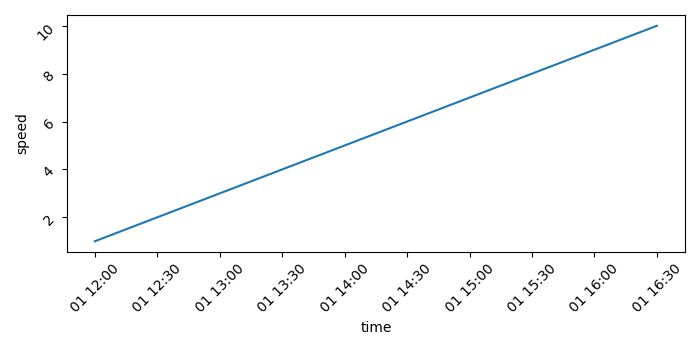# How to plot a time series graph using Seaborn or Plotly?

To plot a time series graph using Seaborn or Plotly, we can take the following steps −

• Set the figure size and adjust the padding between and around the subplots.
• Create a Pandas dataframe, df, to hold a date_time series "time" and another variable data, speed.
• Make a Seaborn line plot with the data, "time" and "speed"
• Rotate the tick params by 45.
• To display the figure, use show() method.

## Example

import seaborn as sns
from matplotlib import pyplot as plt
import pandas as pd
import numpy as np

plt.rcParams["figure.figsize"] = [7.00, 3.50]
plt.rcParams["figure.autolayout"] = True

df = pd.DataFrame(
dict(
time=list(pd.date_range("2021-01-01 12:00:00", periods=10, freq="30min")),
speed=np.linspace(1, 10, 10)
)
)

ax = sns.lineplot(x="time", y="speed", data=df)

ax.tick_params(rotation=45)

plt.show()

## Output

It will produce the following output